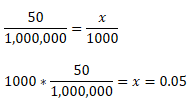# Parts Per Million: Definition, Calculation & Example

Instructor: Julie Waltz
In chemistry we will often see things described as parts per million (ppm), in this lesson we will learn what this means as well as how to calculate ppm.

## What is Parts Per Million?

We are familiar with talking about things as a percentage - such as this hamburger contains 50% of my daily recommended calories. Percent really means parts per hundred, so, similar to percent, is parts per million, only we are usually talking about much smaller concentrations. If we were to refer to 2 parts per million, then this would be 0.0002%, and all of those zeros start to get confusing, so instead, we refer to parts per million for smaller concentrations.

Parts per million (ppm) is how many parts a certain molecule or compound makes up within the one million parts of the whole solution. It is typically used to describe concentrations of chemicals dissolved in a solvent (typically water) or compounds in soil.

## Calculating PPM

So, we mentioned that 2 ppm = 0.0002%, but how would we calculate that? It is fairly simple:

2/1000000 = 0.000002 * 100% = 0.0002%

But, converting ppm in percentage isn't typically very helpful. Instead, we typically are given a ppm for a final solution and need to determine how much of the desired chemical to mix with water, or we are told how much of a certain compound is in soil and need to determine the ppm.

Let's say that we need to make 1 liter of a 50 ppm salt-water solution, how much water and how much salt should we use to make 1 liter? Let's first think about 1 liter as being equal to 1000 mL, we simply need to set up a simple ratio, or setting two things equal to each other, to determine how much salt to use:So for every 1000 mL of solution, we need to use 0.05 g of salt in order to make a 50 ppm salt-water solution. In order to make this solution we would put 50 ppm of salt into a 1 liter container, and then fill the container to the 1 liter line.

To unlock this lesson you must be a Study.com Member.

### Register to view this lesson

Are you a student or a teacher?

### Unlock Your Education

#### See for yourself why 30 million people use Study.com

##### Become a Study.com member and start learning now.
Back
What teachers are saying about Study.com

### Earning College Credit

Did you know… We have over 160 college courses that prepare you to earn credit by exam that is accepted by over 1,500 colleges and universities. You can test out of the first two years of college and save thousands off your degree. Anyone can earn credit-by-exam regardless of age or education level.